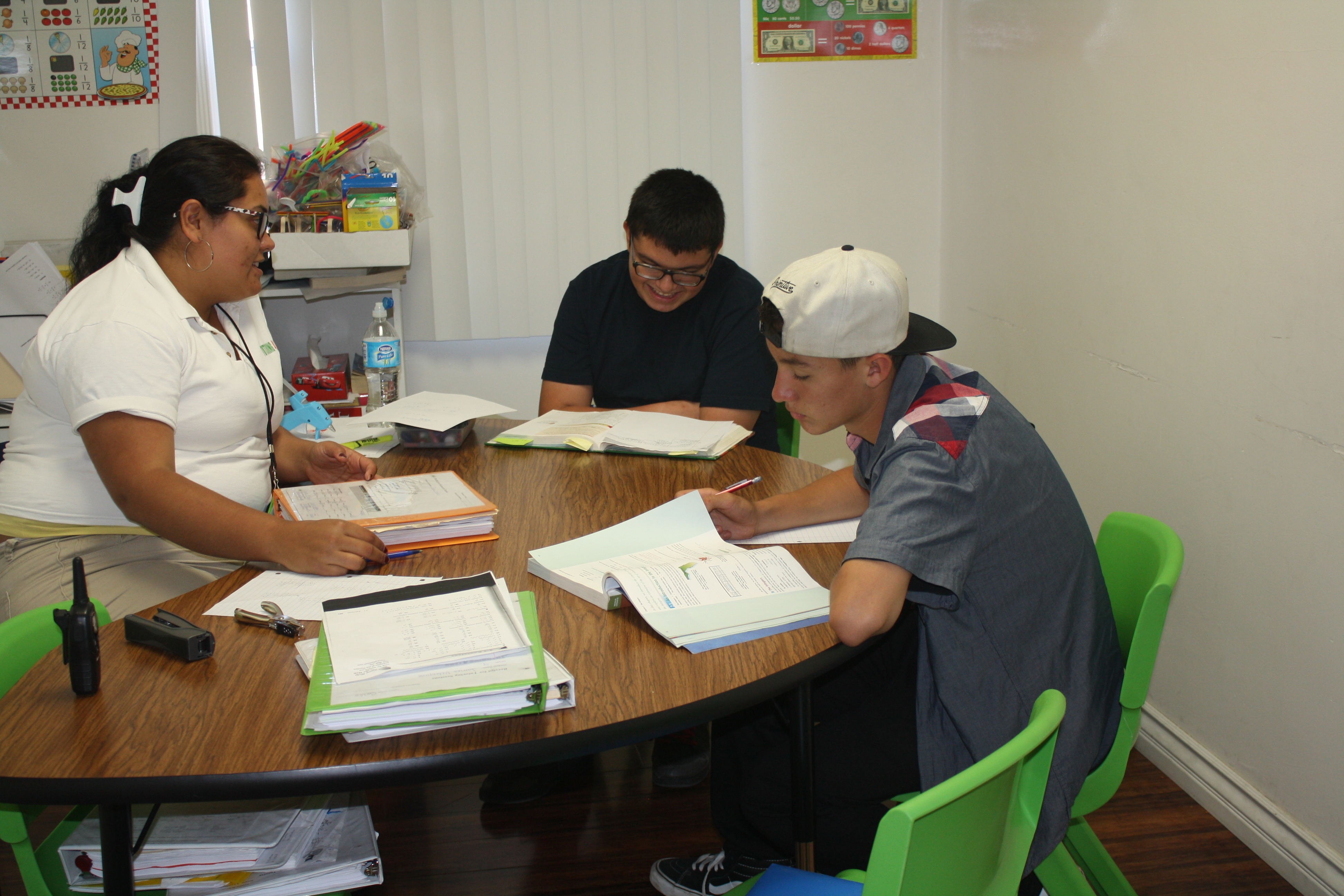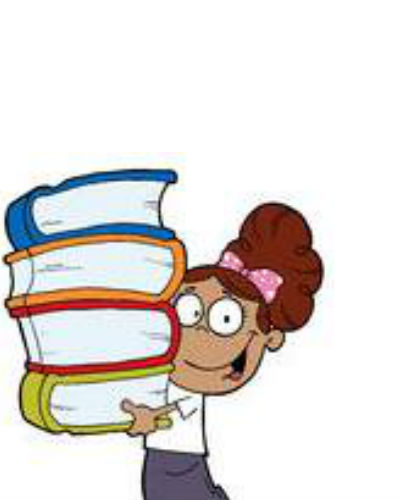#Are you in need of middle school math tutoring for your developing students? Tutoring 4 Less can enhance your student’s foundation of knowledge for a deeper understanding. At Tutoring 4 Less  we strive to prepare each student with the tools that are necessary to become independent learners and to demonstrate a mastery of skills and knowledge in middle school math.  Tutoring 4 Less wants all of our students to recognize the importance of math in the everyday world and to demonstrate confidence and enthusiasm in the classroom.

## Middle School Math Tutors Near Me in Los Angeles

When looking for a middle school math tutor near you in Los Angeles, you may be wondering how to select the best tutor or program for your child. At Tutoring 4 Less, our goal is to provide a successful transition from the basic skills learned in elementary mathematics to the advanced mathematical skills needed to succeed in middle school, and beyond. Most students begin their first transitional year of middle school reviewing and relearning a variety of mathematical concepts to reinforce their understanding in the subject matter. Mastery of the skills at the beginning of their middle school years is essential in preparing students for the subsequent math years. Pre-Algebra, Algebra, Statistics, and Geometry, are learned as a part of an integrated sequence of courses, with each different subject area receiving emphasis each year.## Online Tutoring For Middle School Math

What makes Tutoring 4 Less superior to other online tutoring services for math? We provide more in our hours of tutoring than other companies can offer. We do this by starting with a free assessment of each student at the outset to determine their learning style. When this has been established, we can build a curriculum that plays to each student’s strengths and helps them patch their weaknesses. Under our tutelage, our students typically improve their abilities by an entire grade level, owing to our customized online tutoring curriculum and encouragement towards advanced goals.

Our math tutors in Los Angeles, CA have tutored students of all ages in math, sciences, and all sorts of subjects for years. Especially when handling more advanced math concepts such as the kind students begin to encounter in middle school, the difficulty curve can be punishing. Private tutoring can make the difference between a failed class and an A. Our online programs deliver our proven middle school math programs in a virtual environment, whether your child is currently attending school in-person, virtually, or via a hybrid program. You can feel confident in your middle schooler’s math program with our consistent and professional online tutoring program.

## What Math Classes Do You Take in Middle School?

By the end of their middle school years students are expected to be advanced in algebraic notation and reasoning. They should understand how to integrate algebraic concepts and skills with other advanced branches of mathematics. At Tutoring 4 Less, we organize these learning goals across five learning branches.

• Numbers and Operations: Our primary focus is to develop computational fluency with fractions, decimals, and integers. Students are well versed in using the order of operations and how to apply it to problem solving. By the end of 8th grade, students should have a well-developed understanding of algebraic ideas and concepts that will stand them in good stead for advanced math courses they will encounter as high school students, such as pre-calculus.
• Problem Solving: Students will be able to make sense of problems and be proficient in explaining and analyzing their meaning. They should be comfortable enough to make conjectures about the form and meaning of the problems and plan a solution pathway rather than just memorize steps and jump into guessing a solution. In most cases, they use their knowledge of algebraic expressions and use linear equations to solve the problems. The ultimate goal of this branch of middle school mathematics is to get students to explain the correlation between equations, verbal descriptions, tables, and graphs.
• Geometry and Measurement: This area is focused on heavily during middle school because it is vitally important. This branch in the curriculum is directly linked to the ideas learned in all other areas of middle school mathematics, especially algebra and statistics. Students learn about conversions, units, and different objects; both planar and three-dimensional. Students expand on their ability to calculate area, volumes, and perimeters first developed in elementary school. Sixth grade level math students build on their knowledge of area by reasoning about relationships among shapes to determine area, surface area, and volume. They find areas of right triangles, other triangles, and special quadrilaterals by decomposing these shapes, rearranging or removing pieces, and relating the shapes to rectangles. Using these methods, students discuss, develop, and justify formulas for areas of triangles and parallelograms. Students find areas of polygons, and surface areas of prisms and pyramids by decomposing them into pieces whose area they can determine. They prepare for work on scale drawings and constructions in grade 7 by drawing polygons in the coordinate plane.
• Quantities and Relationships: Students in middle school are introduced to rates and ratios. They are expected to not only solve rate and ratio problems graphically and algebraically, but are encouraged to use the learned concepts and terminology to describe the relationships between the compared quantities. Students in middle school are also introduced to negative integers for the first time. They are expected to understand that positive and negative numbers are used together to describe quantities having opposite directions or values (e.g., temperature above/below zero, elevation above/below sea level, credits/debits, positive/negative electric charge). Students use positive and negative numbers to represent quantities in real-world contexts, explaining the meaning of 0 in each situation.
• Algebra: Algebra is the branch of mathematics that focuses on representing numbers and quantities through variables (letters). Students learn to manipulate symbols and equations to build tables, models, and graphs to outline real world applications. They understand numbers, ways of representing numbers, relationships amongst numbers, and number systems. Algebra reinforces the ability to reason and represent ideas in various forms. Students are encouraged to look for patterns to solve a range of problems by gathering and organizing data to make generalizations about mathematical concepts. They are also taught to analyze mathematical situations and structures using algebraic symbols and functions.